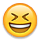# If THEN ELSE syntaxHello, I am having an issue with IF THEN ELSE forumla syntax. I have the following fuctioning forumla, in the context of converting weeks to months. IF Start Difference <= -23 AND Start Difference >= -28 AND End Difference >= 7 THEN ITEM(Week) ELSE BLANK I am trying to add a condition that says if the week start date is a hard date, then also return the ITEM(Week) date.  Neither of the forumlas below are  working.  Does anyone have a suggestion on how I should be adding the Period Start nested formula?  Thanks for any help!! IF Period Start = 1/1/2017 THEN "Week 5" ELSE IF Start Difference <= -23 AND Start Difference >= -28 AND End Difference >= 7 THEN ITEM(Week) ELSE BLANK IF Period Start = 1/1/2017 THEN ITEM(Week) ELSE IF Start Difference <= -23 AND Start Difference >= -28 AND End Difference >= 7 THEN ITEM(Week) ELSE BLANK

Tagged:

•I figured it out, it was the date versus number format.  I converted the day and month to numbers instead of dates and it worked.

IF MONTH(Period Start) = 1 AND MONTH(Week Start) = 1 AND DAY(Week Start) = 30 then 7 ELSE END(ITEM(Time)) - Week Start
•Hi Denise,
I see this is your first post. Welcome to the ForumsI'm not sure I fully understand the underlying problem that you are trying to solve, if possible could you send a screenshot of the module you are working in and the expected result of the forumla? Are Period Start, Start Difference, End Difference other Line items in your module?
Are you using Anaplan's TIME list, or a seperate General List called "Week"?

If you are using Anaplan's built in time scale, in-case you don't know about it check out the Period function, it's really useful. https://community.anaplan.com/anapedia/calculation-functions/period

It looks like you are returning Text ("Week 5") so you would also need to think about using the NAME() function around your ITEM() function. e.g. NAME(ITEM(Week)) if you are trying to return the formula result into a Text Line item. If it is List formatted then you need to change the "Week 5" in your formula to Week.'Week 5', this will return the valid Week 5 item in the Week list.

Hope that helps.
Cheers, Simon